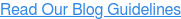### Blog: Subscribe NowFeatured Podcast

Listen Now

# Physical Math Manipulatives vs. Digital Manipulatives

Math manipulatives are objects that allow learners to interact with mathematical concepts. These include everything from cuisenaire rods, legos, blocks, magnets...anything that gets students touching, moving, and experiencing math concepts.

The drawbacks of using these physical math manipulatives in the classroom?

• The teacher needs to be available to give informative feedback.
• It can take a long time to set up and solve a problem with large numbers or exponential concepts.
• If students get used to thinking about fractions as a pizza slice, they may have difficulty transferring that knowledge to other representations.

That's not to say that they shouldn't be used. Research suggests that learning with our bodies lessens the cognitive load and makes learning concepts easier.

Plus, the right virtual manipulatives can supplement and build upon learning from physical manipulatives.

Technology has the capability to give immediate, informative feedback tailored to the student's answer. It can deal with large numbers as quickly as small numbers. And, technology can help provide many different representations of the same math concept, without having to physically dig through boxes of materials.

## Scaffolding with Math Manipulatives

What would it look like if educators used both physical and digital manipulatives to scaffold the concept and improve deeper understanding and mastery of the concept?ST Math®—created by neuroscientists, mathematicians and educational innovators at MIND Research Institute—is a visual math program that builds a deep conceptual understanding of math through rigorous learning and creative problem solving.

Here are some examples of how the ST Math: K-6 program optimizes virtual manipulatives for math comprehension. ST Math’s virtual puzzles and informative feedback gives students the opportunity to make meaning and visualize math concepts as the result of their direct actions, just like any physical manipulative.

Let’s take a look at some of these interactive puzzles and how they compare to other visual models and physical manipulatives often used in mathematics classrooms.

### Visual Patterns

In early learning classrooms, students are often asked to draw the next shape of a given pattern.Visual software guides students through a rich experience building patterns from start to finish.Visual Patterns in ST Math: Early Learning

### Place Value Blocks

Place value blocks are a great way to build conceptual understanding of base 10.This ST Math puzzle asks students how many blocks they’ll need to add to make 100 total.Place Value Bundles game in second grade, ST Math: K-6.

### Place Value Charts

Place Value Mats help students connect words and symbols to corresponding quantities.ST Math gives students the opportunity to make connections between symbols to words and back to symbols.3-Digit Number Words game in second grade, ST Math: K-6.

### Number Line Jumps

Teachers can teach basic operations with a number line.Interactive software will allow the exact same experience with precise, quick feedback.Number Line Jumps game in second grade, ST Math: K-6.

### Area Models

Conceptual instruction on multi-digit multiplication often relies on place value area models.With a virtual manipulative, students can see how the area model directly connects to the multiplication algorithm.Multi-digit multiplication game in  fourth grade, ST Math: K-6.

### Fraction Circular Models

Circular models are an intuitive way to show fraction conceptsWith a virtual manipulative, students can easy use circular models for all fraction operations.Fraction Multiples game in fourth grade, ST Math: K-6.

### Fraction Area Models

To solve problems with area models, students usually have to draw or cut them out.Visual software allows students the opportunity to quickly manipulate an area model and see the feedback immediately.Fraction Multiplication game in sixth grade, ST Math: K-6.

Algebra tiles offer a powerful conceptual model for complex polynomial operations.With ST Math, students experience algebra tiles with immediate, informative feedback showing exactly why their answer is correct or incorrect.Unlike static manipulatives, where feedback has to come from other students or teachers, in ST Math students are able to see and interact with abstract math concepts through graphically rich digital manipulatives and receive real-time, informative feedback. ST Math’s rigorous puzzles facilitate students in action-oriented learning, increasing problem-solving skills.